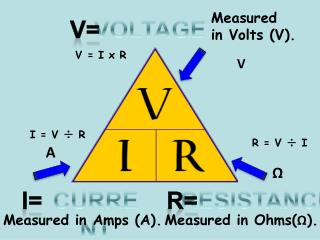DownloadDownload PresentationCURRENT

# CURRENT

Télécharger la présentation## CURRENT

- - - - - - - - - - - - - - - - - - - - - - - - - - - E N D - - - - - - - - - - - - - - - - - - - - - - - - - - -
##### Presentation Transcript

1. Measured in Volts (V). V= VOLTAGE V = I x R V I = V ÷ R R = V ÷ I A Ω I= RESISTANCE CURRENT R= Measured in Amps (A). Measured in Ohms(Ω).

2. If you know the current, (i) and the resistance (r), then V=IR • If you know the voltage(V) and the resistance (r) then I = V/R • If you know the current (i) and the voltage (v) then R=VI